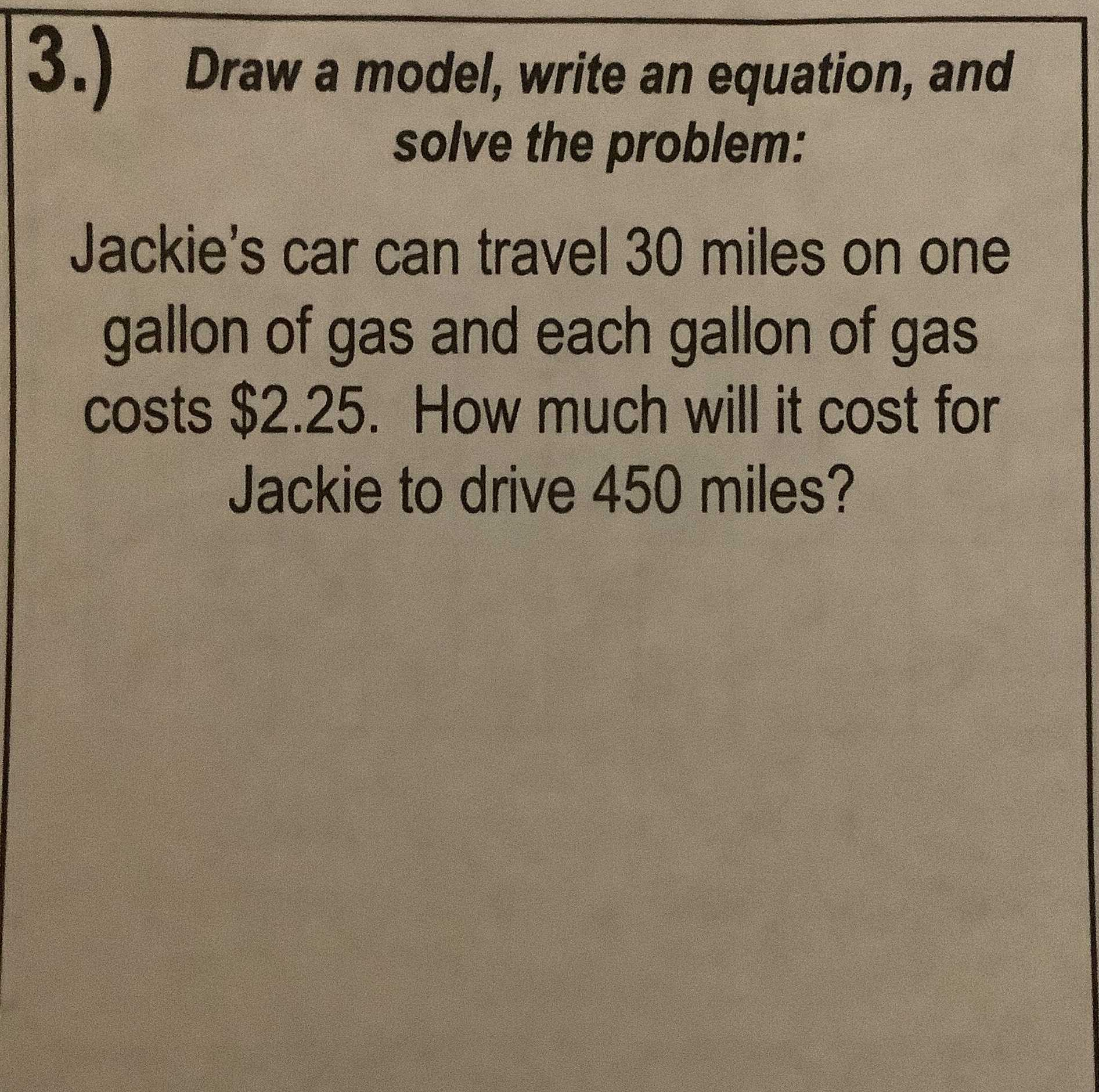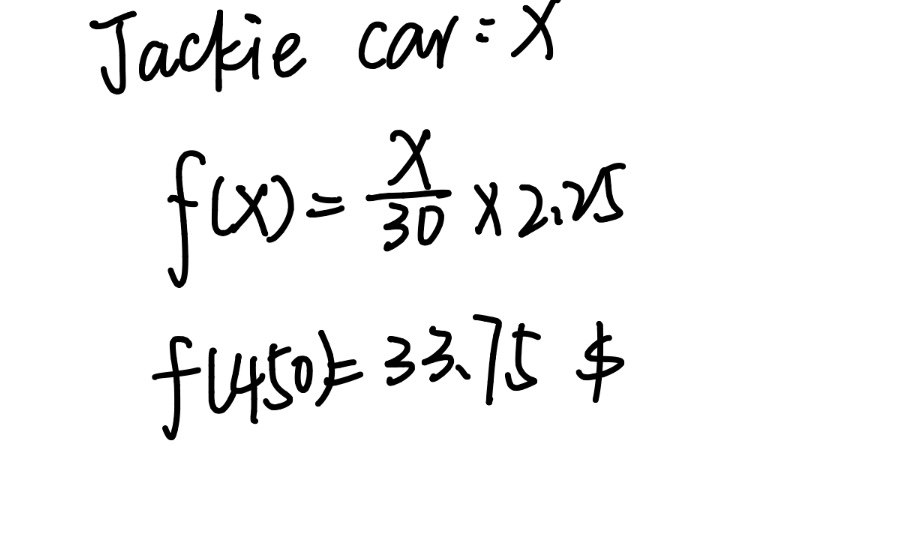### ¿Todavía tienes preguntas de matemáticas?

Pregunte a nuestros tutores expertos
Algebra
Pregunta3.) Draw a model, write an equation, and solve the problem: Jackie's car can travel $$30$$ miles on one gallon of gas and each gallon of gas costs $$\ 2.25$$ . How much will it cost for Jackie to drive $$450$$ miles?